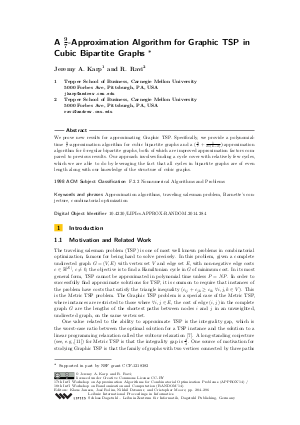Document# A 9/7 -Approximation Algorithm for Graphic TSP in Cubic Bipartite Graphs

### Authors Jeremy A. Karp, R. Ravi## File

LIPIcs.APPROX-RANDOM.2014.284.pdf
• Filesize: 0.51 MB
• 13 pages

## Cite As

Jeremy A. Karp and R. Ravi. A 9/7 -Approximation Algorithm for Graphic TSP in Cubic Bipartite Graphs. In Approximation, Randomization, and Combinatorial Optimization. Algorithms and Techniques (APPROX/RANDOM 2014). Leibniz International Proceedings in Informatics (LIPIcs), Volume 28, pp. 284-296, Schloss Dagstuhl - Leibniz-Zentrum für Informatik (2014)
https://doi.org/10.4230/LIPIcs.APPROX-RANDOM.2014.284

## Abstract

We prove new results for approximating Graphic TSP. Specifically, we provide a polynomial-time 9/7-approximation algorithm for cubic bipartite graphs and a (9/7+1/(21(k-2)))-approximation algorithm for k-regular bipartite graphs, both of which are improved approximation factors compared to previous results. Our approach involves finding a cycle cover with relatively few cycles, which we are able to do by leveraging the fact that all cycles in bipartite graphs are of even length along with our knowledge of the structure of cubic graphs.
##### Keywords
• Approximation algorithms
• traveling salesman problem
• Barnette’s conjecture
• combinatorial optimization

## Metrics

• Access Statistics
• Total Accesses (updated on a weekly basis)
0